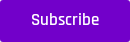Classification

# Support Vector Classifier Simply Explained [With Code]

Let's take a deep dive & try to understand Support Vector Classifier, a popular supervised machine learning classification algorithm with the help of code

Support Vector Machine (SVM) is a supervised machine learning algorithm that has become immensely popular in solving classification as well as regression problems.

It was initially introduced to address binary classification problems but with time, it was extended to include regression analysis as well owing to its robustness.

The dataset used contains a list of features such as job, employment history, housing, marital status, purpose, savings, etc. to predict whether a customer will be able to pay back a loan.

The target variable is ‘good_bad’. It consists of two categories: good and bad. Good means the customer will be able to repay the loan, whereas bad means the customer will default. It is a binary classification problem meaning the target variable consists of two classes only.

This is a very common use case in banks and financial institutions, as they have to decide whether to give a loan to a new customer based on how likely it is that the customer will be able to repay it.

## SVC: Background Knowledge

The SVM classifier iteratively constructs hyperplanes to learn a decision boundary in order to separate data points that belong to different classes. The hyperplanes are chosen to

• maximize the distance of the decision boundary to support vectors

• maximize the number of points that are correctly classified in the training set

If our data can be perfectly separated using a hyperplane, then that implies that there exists an infinite number of hyperplanes that could achieve the purpose. So which hyperplane should be chosen? The problem can be illustrated by taking a look at the figure below where we can draw infinitely many lines (like the ones in yellow and black)  to separate the two classes marked in red and green.Here comes the concept of soft margin vs hard margins (a margin is the distance between the line and the closest data point of the classes). The optimal hyperplane according to a hard margin would minimize the distance between the data points and maximize the decision boundary as can be seen in the figure below.Figure: Hard Margin Classifier

The margin is calculated as the perpendicular distance from the line to only the closest points. These points are called the support vectors as they support or define the hyperplane. The hyperplane is learned from the training data using an optimization procedure that maximizes the margin.

In real-world scenarios, it is not possible to achieve perfect separation with a hyperplane. There will always be some misclassified instances. This phenomenon is incorporated in soft margin which allows some data points to be on the wrong side of the margin or even on the wrong side of the hyperplane as shown in the figure below:Figure: Soft Margin Classifier

The data point x1 is on the wrong side of its margin but on the right side of the hyperplane, whereas the data point x2 is not only on the wrong side of its margin but also on the wrong side of the hyperplane. These two points along with x3, x4 and x5, which lie exactly on the soft margin, are called the support vectors.

## Hyperparameters for SVM

### 1. C

The softness of the margins is controlled by a parameter called ‘C’. It is a regularisation parameter that controls the trade-off between decision boundary and misclassification term. The higher the value of C, the harder would be the margin, and more data points tend to be correctly classified (see the figure below). However, a higher C value also causes the model to overfit.  Lower the value of C, softer would be the margin and the misclassification increases but the model tends to generalize well on unseen data.Figure: Different values of C and the resulting decision boundary and the misclassification

### 2. Gamma

Another hyperparameter of interest for non-linear data is gamma, which determines the amount of curvature in a decision boundary. It determines how far the influence of a single training example reaches, with low values meaning ‘far’ and high values meaning ‘close’. The figure below would help illustrate this point better.### 3. Kernels

SVM works extremely well when handling high dimensional datasets. It does this by constructing a hyperplane that separates the data in space which we might not be able to do. In the worst case scenario, the data might even not be linearly separable. The hyperplanes can be of different types where linear planes are of the lowest complexity. If the data is not separable in space, a technique known as kernelling is applied which maps the observations from a general space to a high dimensional feature space where we know the data is linearly separable. There are multiple standard kernels, e.g. the linear kernel, the polynomial kernel and the radial kernel. The choice of the kernel and their hyperparameters greatly affect the separability of the classes (in classification) and the performance of the algorithm.## Python Implementation

We have implemented Support Vector Classifier in Python for the following kernels:

• Linear

• RBF

• Polynomial

• Sigmoid

### Wrap-Up

This blog aimed to give you an overview of Support Vector Classifiers. The concept of hard vs soft margins was explained followed by a discussion on the hyperparameters for SVC. The algorithm was then implemented in Python using different kernels and hyper-parameter optimization was carried out. Interested in the fundamentals of Machine Learning? Read this article to learn more!

### Interested and want to learn more? Simply subscribe to our weekly emails for a whole new world of information!